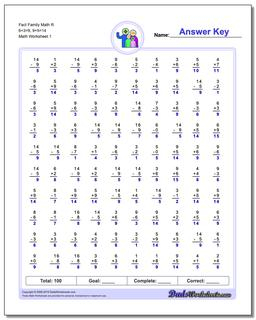# Math Worksheets: Fact Family Math: Fact Family Math: Fact Family Math R 6+3=9, 9+5=14## Fact Family Math R 6+3=9, 9+5=14

PropertyValue
DescriptionFact Family Math R 6+3=9, 9+5=14: These math worksheets have 100 addition and subtraction fact family problems and make for a challenging two minute test.
Resource TypeWorksheet